20.2
20.1
19.2
19.1
18.2
18.1
17.2
.NET Framework 4.5.2+
.NET Framework 4.5.2+
.NET Standard 2.0+

# Dates and Times in Cells

Dates and times are stored in cells as numbers. Thus, the values of cells that contain dates and times are of the numeric type. A number that specifies a date and time consists of the date (integer part) and time (fractional part) components. The CellValue.NumericValue property returns this number.

To display a number as a date and time, apply the required date and time format to a cell via the Formatting.NumberFormat property. The CellValue.DateTimeValue property returns the DateTime object, which specifies the date and time that is represented by the number contained in a cell.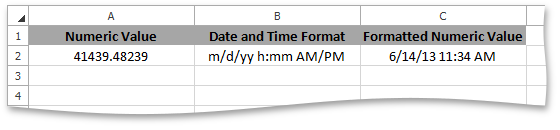To obtain whether or not a cell displays its numeric value as date and time, use the Cell.IsDisplayedAsDateTime property.

## Dates in Cells

SpreadsheetControl stores dates as numbers that are called serial values.

A serial value is an integer that is the number of elapsed days from the first day in the date system. SpreadsheetControl supports the following date systems for serial values:

• The 1900 date system. The first date is January 1, 1900, and its serial value is 1. The last date is December 31, 9999, and its serial value is 2,958,465.

This date system is used in the workbook by default.

• The 1904 date system. The first date is January 1, 1904, and its serial value is 0. The last date is December 31, 9999, and its serial value is 2,957,003.

To use this date system in the workbook, set the Workbook.DocumentSettings.Calculation.Use1904DateSystem property to true.

The serial value of the date contained in a cell is the integer part of the number that the CellValue.NumericValue property returns. You can input a date into a cell in one of the following ways:

• Set the CellRange.Value property to a number that specifies the date's serial value in the 1900 or 1904 date system.
• Set the CellRange.Value property to a DateTime object. The date's serial value will be calculated in the 1900 date system.
• Set the CellRange.Value property to an object that is returned by the CellValue.FromDateTime method. The date's serial value will be calculated based on the passed use1904DateSystem parameter value.
• Set the CellRange.Formula property to an expression that returns a date. The date's serial value will be calculated according to the date system used in the workbook.

To obtain the date that corresponds to the serial value contained in a cell, use the CellValue.DateTimeValue property. This date is defined according to the date system used in the workbook. In other words, the CellValue.DateTimeValue property specifies the date that will be displayed in a cell when you apply a date format.

For example, consider the 12/17/2012 date. The table below demonstrates which serial values this date is represented by in each date system, how the date can be assigned to a cell in each date system, and how the date displayed depends on the date system applied in the workbook.

Date System to Input Date

Serial Value of 12/17/2012

Input Date

Use1904DateSystem = false

Use1904DateSystem = true

1900

41260

cell.Value = new DateTime(2012, 12, 17)

CellValue.NumericValue = 41260

CellValue.DateTimeValue = {12/17/2012 12:00:00 AM}

CellValue.NumericValue = 41260

CellValue.DateTimeValue = {12/18/2016 12:00:00 AM}

1904

39798

cell.Value = CellValue.FromDateTime(new DateTime(2012, 12, 17), true);

CellValue.NumericValue = 39798

CellValue.DateTimeValue = {12/16/2008 12:00:00 AM}

CellValue.NumericValue = 39798

CellValue.DateTimeValue = {12/17/2012 12:00:00 AM}

## Times in Cells

SpreadsheetControl stores times as decimal fractions that range from 0 to 0.99999, which represent the time range from 12:00:00 AM to 11:59:59 PM. The cell's time value is the fractional part of the number that the CellValue.NumericValue property returns.

To obtain the time that corresponds to the decimal fraction of the cell's CellValue.NumericValue number, use the CellValue.DateTimeValue property. This time will be displayed in a cell when you apply a date format.

## Date and Time Display Formats

To display a number as a date and time, associate the appropriate display format with the cell via the Formatting.NumberFormat property. Use the CellRange.BeginUpdateFormatting-CellRange.EndUpdateFormatting method pair to access and modify the Formatting.NumberFormat for the cell range. To apply a number format to a single cell, use the cell's NumberFormat property directly. Refer to the How to: Specify Number or Date Format for Cell Content example.

The Cell.DisplayText property returns the value as it is displayed in a cell.

##### NOTE

If a number is negative or excessively large (greater than the last date in the date system used), it will be displayed as ##### in the cell when you apply the date and time format.

When you format date and time values in a cell, you can specify display formats that rely on the operating system's regional date and time settings. The table below lists these formats in the Invariant Culture column. When one of the specified formats is applied to a cell, its date and time value displays differently depending on the user's current locale. The last two table columns show how the specified formats are interpreted in the English (United States) ("en-US") and French ("fr-FR") cultures.

Invariant Culture

English Culture

(United States)

French Culture

"mm-dd-yy"

cell.Value = DateTime.Now;

cell.NumberFormat = "mm-dd-yy"

"m/d/yyyy"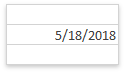"jj/mm/aaaa"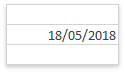"d-mmm-yy"

cell.Value = DateTime.Now;

cell.NumberFormat = "d-mmm-yy"

"d-mmm-yy"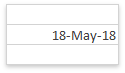"jj-mmm-aa"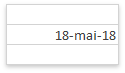"d-mmm"

cell.Value = DateTime.Now;

cell.NumberFormat = "d-mmm"

"d-mmm"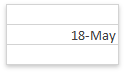"jj-mmm"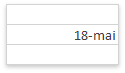"mmm-yy"

cell.Value = DateTime.Now;

cell.NumberFormat = "mmm-yy"

"mmm-yy"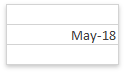"mmm-aa"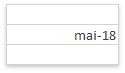"m/d/yy h:mm"

cell.Value = DateTime.Now;

cell.NumberFormat = "m/d/yy h:mm"

"m/d/yyyy h:mm"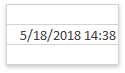"jj/mm/aaaa hh:mm"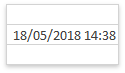"hh:mm AM/PM"

cell.Value = DateTime.Now;

cell.NumberFormat = "hh:mm AM/PM"

"h:mm AM/PM"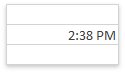"h:mm AM/PM""hh:mm:ss AM/PM"

cell.Value = DateTime.Now;

cell.NumberFormat = "hh:mm:ss AM/PM"

"h:mm:ss AM/PM"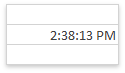"h:mm:ss AM/PM""h:mm"

cell.Value = DateTime.Now;

cell.NumberFormat = "h:mm"

"h:mm"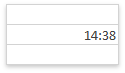"hh:mm""h:mm:ss"

cell.Value = DateTime.Now;

cell.NumberFormat = "h:mm:ss"

"h:mm:ss"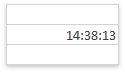"hh:mm:ss"